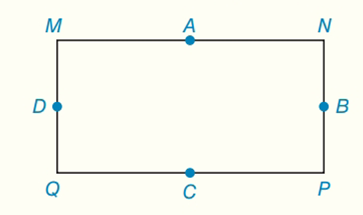Chapter 7.CT, Problem 16CTElementary Geometry For College St...

7th Edition
Alexander + 2 others
ISBN: 9781337614085

Solutions

Chapter
SectionElementary Geometry For College St...

7th Edition
Alexander + 2 others
ISBN: 9781337614085
Textbook Problem

For rectangle MNPQ, points A, B, C and D are the midpoints of the sides.a) What type of quadrilateral (not shown) is ABCD?b) Are the inscribed circle for ABCD and the circumscribed circle for MNPQ concentric circles?To determine

(a)

To find:

ABCD is what type of quadrilateral.

Explanation

Properties of rhombus:

1. All sides are congruent.

2. Diagonals are perpendicular bisectors of each other.

3. Each diagonal is the angle bisector of both the opposite angles.

4. Every rhombus is a parallelogram and a kite with all sides of equal length.

Calculation:

Given:

For rectangle MNPQ, points A, B, C and D are the midpoints of the sides.

Now we have to what type of quadrilateral (not shown) is ABCD...

To determine

(b)

To find:

The inscribed circle for ABCD and the circumscribed circle for MNPQ are concentric circles.

Still sussing out bartleby?

Check out a sample textbook solution.

See a sample solution

The Solution to Your Study Problems

Bartleby provides explanations to thousands of textbook problems written by our experts, many with advanced degrees!

Get Started